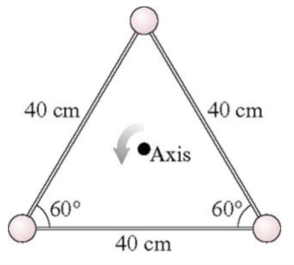# Problem: The three 190 g masses in the figure (Figure 1) are connected by massless, rigid rods.(a) What is the triangle's moment of inertia about the axis through the center? Express your answer to two significant figures and include the appropriate units.(b) What is the triangle's kinetic energy if it rotates about the axis at 4.4 rev/s? Express your answer to two significant figures and include the appropriate units.

###### FREE Expert Solution

Moment of inertia:

$\overline{){\mathbf{I}}{\mathbf{=}}{\mathbf{m}}{{\mathbf{r}}}^{{\mathbf{2}}}}$

I = mr2

Kinetic energy:

$\overline{){\mathbf{K}}{\mathbf{.}}{\mathbf{E}}{\mathbf{=}}\frac{\mathbf{1}}{\mathbf{2}}{\mathbf{I}}{{\mathbf{\omega }}}^{{\mathbf{2}}}}$

(a)

I = mr2 + mr2 + mr= 3mr2

r is the distance from the center to the vertex.

94% (388 ratings)###### Problem Details

The three 190 g masses in the figure (Figure 1) are connected by massless, rigid rods.

(a) What is the triangle's moment of inertia about the axis through the center? Express your answer to two significant figures and include the appropriate units.
(b) What is the triangle's kinetic energy if it rotates about the axis at 4.4 rev/s? Express your answer to two significant figures and include the appropriate units.